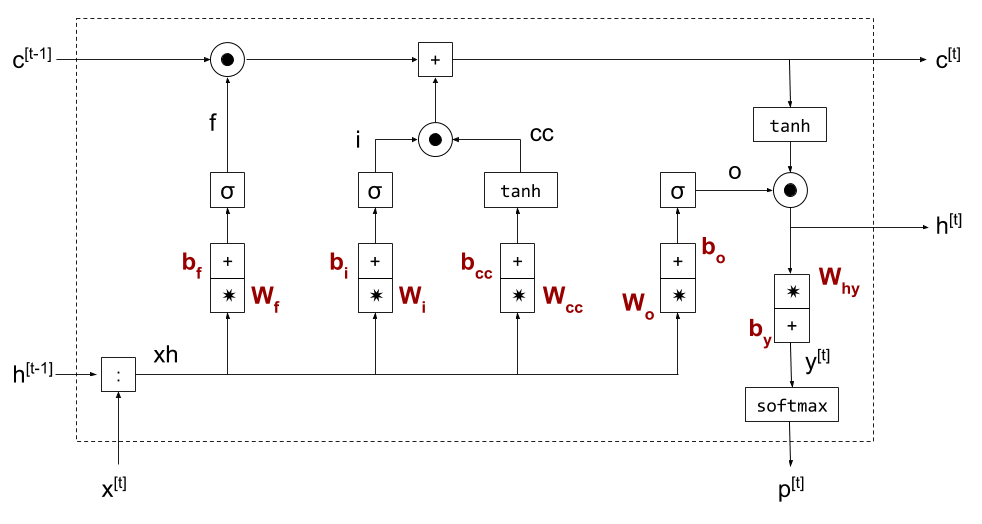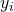Original post

Following up on the earlier post
deciphering a minimal vanilla RNN implementation, here I’d like to extend the
example to a simple LSTM model.

Once again, the idea is to combine a well-commented code sample
(available here)
with some high-level diagrams and to enable someone to
fully understand the code. The LSTM architecture presented herein is the
standard one originating from Hochreiter’s and Schmidthuber’s 1997 paper. It’s described pretty much
everywhere; Chris Olah’s post has particularly
nice diagrams and is worth reading.

## LSTM cell structure

From 30,000 feet, LSTMs look just like regular RNNs; there’s a “cell” that has a
recurrent connection (output tied to input), and when trained this cell is
usually unrolled to some fixed length.

So we can take the basic RNN structure from the previous post:LSTMs are a bit trickier because there are two recurrent connections; these
can be “packed” into a single vector h, so the above diagram still applies.
Here’s how an LSTM cell looks inside:x is the input; p is the probabilities computed from the output y (these
symbols are named consistently with my earlier RNN post) and exit the cell at
the bottom purely due to topological convenience. The two memory vectors are h
and c – as mentioned earlier, they could be combined into a single vector, but
are shown here separately for clarity.

The main idea of LSTMs is to enable training of longer sequences by providing
a “fast-path” to back-propagate information farther down in memory. Hence the
c vector is not multiplied by any matrices on its path. The circle-in-circle
block means element-wise multiplication of two vectors; plus-in-square is
element-wise addition. The funny greek letter is the Sigmoid non-linearity:

[sigma(x) =frac{1}{1+e^{-x}}]

The only other block we haven’t seen in the vanilla RNN diagram is the
colon-in-square in the bottom-left corner; this is simply the concatenation of
h and x into a single column vector. In addition, I’ve combined the
“multiply by matrix W, then add bias b” operation into a single rectantular
box to save on precious diagram space.

Here are the equations computed by a cell:

[begin{align*}
xh&=x^{[t]}:h^{[t-1]}
f&=sigma(W_fcdot xh+b_f)
i&=sigma(W_icdot xh+b_i)
o&=sigma(W_ocdot xh+b_o)
cc&=tanh(W_{cc}cdot xh+b_{cc})
c^{[t]}&=c^{[t-1]}odot f +ccodot i
h^{[t]}&=tanh(c^{[t]})odot o
y^{[t]}&=W_{y}cdot h^{[t]}+b_y
p^{[t]}&=softmax(y^{[t]})
end{align*}]

## Backpropagating through an LSTM cell

This works exactly like backprop through a vanilla RNN; we have to carefully
compute how the gradient flows through every node and make sure we properly
combine gradients at fork points. Most of the elements in the LSTM diagram are
familiar from the previous post.
Let’s briefly work through the new ones.

First, the Sigmoid function; it’s an elementwise function, and computing its
derivative is very similar to the tanh function discussed in the previous
post. As usual, given f=sigma(k), from the chain rule we have the
following derivative w.r.t. some weight w:

[frac{partial f}{partial w}=frac{partial sigma(k)}{partial k}frac{partial k}{partial w}]

To compute the derivative frac{partial sigma(k)}{partiak k}, we’ll
use the ratio-derivative formula:

[(frac{f}{g})’=frac{f’g-g’f}{g^2}]

So:

[sigma ‘(k)=frac{e^{-k}}{(1+e^{-k})^2}]

A clever way to express this is:

[sigma ‘(k)=sigma(k)(1-sigma(k))]

Going back to the chain rule with f=sigma(k), we get:

[frac{partial f}{partial w}=f(1-f)frac{partial k}{partial w}]

The other new operation we’ll have to find the derivative of is element-wise
multiplication. Let’s say we have the column vectors x, y and z, each with
m rows, and we have z(x)=xodot y. Since z as a function of x
has m inputs and m outputs, its Jacobian has dimensions [m,m].

D_{j}z_{i} is the derivative of the i-th element of z w.r.t. the j-th
element of x. For z(x)=xodot y this is non-zero only
when i and j are equal, and in that case the derivative is.

Therefore, Dz(x) is a square matrix with the elements of y on the
diagonal and zeros elsewhere:

[Dz=begin{bmatrix}
y_1 & 0 & cdots & 0
0 & y_2 & cdots & 0
vdots & ddots & ddots & vdots
0 & 0 & cdots & y_m
end{bmatrix}]

If we want to backprop some loss L through this function, we get:

[frac{partial L}{partial x}=frac{partial L}{partial z}Dz]

As x has m elements, the right-hand side of this equation multiplies a [1,m]
vector by a [m,m] matrix which is diagonal, resulting in element-wise multiplication
with the matrix’s diagonal elements. In other words:

[frac{partial L}{partial x}=frac{partial L}{partial z}odot y]

In code, it looks like this:

```# Assuming dz is the gradient of loss w.r.t. z; dz, y and dx are all
# column vectors.
dx = dz * y
```

## Model quality

we’ve seen that the vanilla RNN generates fairly low quality text:

one, my dred, roriny. qued bamp gond hilves non froange saws, to mold
his a work, you shirs larcs anverver strepule thunboler
muste, thum and cormed sightourd
so was rewa her besee pilman

The LSTM’s generated text quality is somewhat better when trained with roughtly
the same hyper-parameters:

the she, over is was besiving the fact to seramed for i said over he
will round, such when a where, “i went of where stood it at eye heardul rrawed
only coside the showed had off with the refaurtoned

I’m fairly sure that it can be made to perform even better with larger memory
vectors and more training data. That said, an even more advanced architecture
can be helpful here. Moreover, since this is a character-based model, to
really capture effects between words a few words apart we’ll need a much deeper
LSTM (I’m unrolling to 16 characters we can only capture 2-3 words), and hence
much more training data and time.

Once again, the goal here is not to develop a state-of-the-art language model,
but to show a simple, comprehensible example of how and LSTM is implemented
end-to-end in Python code. The full code is here
– please let me know if you find any issues with it or something still remains
unclear.